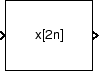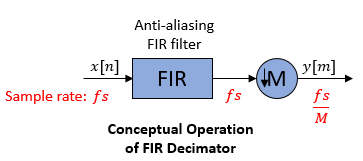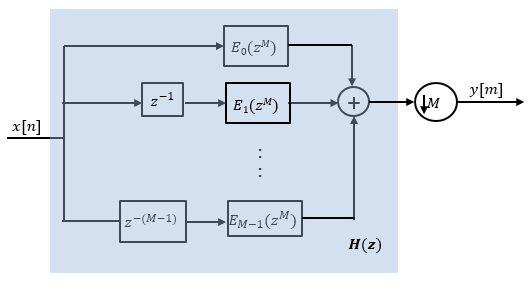# FIR Decimation

Filter and downsample input signals

• Library:
• DSP System Toolbox / Filtering / Multirate Filters

DSP System Toolbox HDL Support / Filtering

•## Description

The FIR Decimation block resamples vector or matrix inputs along the first dimension. The FIR decimator (as shown in the schematic) conceptually consists of an anti-aliasing FIR filter followed by a downsampler. To design an FIR anti-aliasing filter, use the `designMultirateFIR` function.

The FIR filter filters the data in each channel of the input using a direct-form FIR filter. The downsampler that follows downsamples each channel of filtered data by taking every M-th sample and discarding the M – 1 samples that follow. M is the value of the decimation factor that you specify. The resulting discrete-time signal has a sample rate that is 1/M times the original sample rate.The actual block algorithm implements a direct-form FIR polyphase structure, an efficient equivalent of the combined system depicted in the diagram. For more details, see Algorithms.

Under specific conditions, this block also supports SIMD code generation. For details, see Code Generation.

## Ports

### Input

expand all

Specify the data input as a vector or a matrix.

When the Rate options parameter is set to `Enforce single-rate processing`, the number of rows in the input must be a multiple of the Decimation factor parameter.

This block supports variable-size inputs. That is, the frame size (number of rows) of the signal can change during simulation but the number of channels cannot.

This port is unnamed until you set Coefficient source to Input port.

Data Types: `single` | `double` | `int8` | `int16` | `int32` | `uint8` | `uint16` | `uint32` | `fixed point`
Complex Number Support: Yes

Specify the numerator coefficients of the FIR filter as a vector.

The transfer function H(z) of the FIR filter is given by:

`$H\left(z\right)={b}_{0}+{b}_{1}{z}^{-1}+...+{b}_{N}{z}^{-N}$`

You can generate the FIR filter coefficient vector, b = [b0, b1, …, bN], using one of the DSP System Toolbox™ filter design functions such as `designMultirateFIR`, `firnyquist`, `firhalfband`, `firgr`, or `firceqrip`.

To act as an effective anti-aliasing filter, the coefficients usually correspond to a lowpass filter with a normalized cutoff frequency no greater than 1/M, where M is the decimation factor. To design such a filter, use the `designMultirateFIR` function.

Coefficient values obtained through Num are tunable, that is, they can change during simulation, while their properties must remain constant.

The data type of the Num input must match the data type of the In input.

#### Dependencies

The Num input port appears when you set Coefficient source as Input port.

Data Types: `single` | `double` | `int8` | `int16` | `int32` | `uint8` | `uint16` | `uint32` | `fixed point`
Complex Number Support: Yes

### Output

expand all

Output of the FIR Decimator block, returned as a vector or a matrix.

When Rate options is set to:

• `Enforce single-rate processing` — The block maintains the input sample rate and decimates the signal by decreasing the output frame size by a factor of M.

• `Allow multirate processing` — The block decimates the signal such that the output sample rate is M times slower than the input sample rate.

This port is unnamed until you set Coefficient source to Input port.

Data Types: `single` | `double` | `int8` | `int16` | `int32` | `uint8` | `uint16` | `uint32` | `fixed point`
Complex Number Support: Yes

## Parameters

expand all

Coefficient source

Specify the FIR filter coefficient source as one of the following:

• Dialog parameters –– Specify the filter coefficients through the FIR filter coefficients parameter in the block dialog box.

• Input port –– Specify the filter coefficients through the Num input port.

• Filter object –– Specify the filter using a `dsp.FIRDecimator` System object™.

• Auto –– When you select Auto, the block designs an FIR decimator using the decimation factor that you specify in Decimation factor. The `designMultirateFIR` function designs the filter and returns the coefficients used by the block.

Main Tab

Specify the lowpass FIR filter coefficients, in descending powers of z, as a vector. By default, `designMultirateFIR(1,2)` computes the filter coefficients.

The transfer function H(z) of the FIR filter is given by:

`$H\left(z\right)={b}_{0}+{b}_{1}{z}^{-1}+...+{b}_{N}{z}^{-N}$`

You can generate the FIR filter coefficient vector, b = [b0, b1, …, bN], using one of the DSP System Toolbox filter design functions such as `designMultirateFIR`, `firnyquist`, `firhalfband`, `firgr`, or `firceqrip`.

To act as an effective anti-aliasing filter, the coefficients usually correspond to a lowpass filter with a normalized cutoff frequency no greater than 1/M, where M is the decimation factor. To design such a filter, use the `designMultirateFIR` function.

The block internally initializes all filter states to zero.

#### Dependencies

This parameter appears only when you set the Coefficient source to Dialog parameters.

Data Types: `single` | `double` | `int8` | `int16` | `int32` | `int64` | `uint8` | `uint16` | `uint32` | `uint64`
Complex Number Support: Yes

Specify the integer factor M. The block decreases the sample rate of the input sequence by this factor.

#### Dependencies

This parameter appears only when you set the Coefficient source to Dialog parameters, Input port, or Auto.

Data Types: `single` | `double` | `int8` | `int16` | `int32` | `int64` | `uint8` | `uint16` | `uint32` | `uint64`

Specify the FIR filter structure as either ```Direct form``` or ```Direct form transposed```.

#### Dependencies

This parameter appears only when you set the Coefficient source to Dialog parameters, Input port, or Auto.

Specify the name of the multirate filter object that you want the block to implement. You must specify the filter as a `dsp.FIRDecimator`System object.

You can define the System object directly in the block dialog box. Alternatively, you can define the object in a MATLAB® workspace variable and specify the variable in the block dialog box.

For information on creating System objects, see Define Basic System Objects.

#### Dependencies

This parameter appears only when you set the Coefficient source to Filter object.

Specify how the block should process the input. You can set this parameter to one of the following options:

• `Columns as channels (frame based)` — When you select this option, the block treats each column of the input as a separate channel.

• `Elements as channels (sample based)` — When you select this option, the block treats each element of the input as a separate channel.

Specify the method by which the block should decimate the input. You can select one of the following options:

• `Enforce single-rate processing` — When you select this option, the block maintains the input sample rate and decimates the signal by decreasing the output frame size by a factor of M. To select this option, you must set the Input processing parameter to ```Columns as channels (frame based)```.

When you set the Rate options parameter to `Enforce single-rate processing`, you can use the FIR Decimation block inside triggered subsystems.

• `Allow multirate processing` — When you select this option, the block decimates the signal such that the output sample rate is M times slower than the input sample rate.

When you set the FIR Decimation block to the frame-based processing mode, the block can exhibit one-frame latency. In the case of one-frame latency, this parameter specifies the output of the block until the first filtered input sample is available. Specify this parameter as a scalar value to be applied to all signal channels, or as a matrix containing one value for each channel.

Cases of one-frame latency can occur when the input frame size is greater than one, and you set the Input processing and Rate options parameters of the FIR Decimation block as follows:

• Input processing set to ```Columns as channels (frame based)```

• Rate options set to ```Allow multirate processing```

For more information on latency in the FIR Decimation block, see Latency.

#### Dependencies

This parameter appears only when you configure the block to perform multirate processing by setting Rate options to `Allow multirate processing`.

Data Types: `single` | `double` | `int8` | `int16` | `int32` | `int64` | `uint8` | `uint16` | `uint32` | `uint64`
Complex Number Support: Yes

Click on this button to open the Filter Visualization Tool (`fvtool`) and display the filter response of the filter defined in the block dialog box.

Data Types Tab

Select the rounding mode for fixed-point operations. The default is `Floor`. The filter coefficients do not obey this parameter and always round to `Nearest`.

Note

The Rounding mode and Saturate on integer overflow settings have no effect on numerical results when all the following conditions exist:

• Product output is ```Inherit: Inherit via internal rule```

• Accumulator is ```Inherit: Inherit via internal rule```

• Output is ```Inherit: Same as accumulator```

With these data type settings, the block is effectively operating in the full-precision mode.

When you select this parameter, the block saturates the result of its fixed-point operation. When you clear this parameter, the block wraps the result of its fixed-point operation. For details on `saturate` and `wrap`, see overflow mode for fixed-point operations.

Note

The Rounding mode and Saturate on integer overflow parameters have no effect on numeric results when all these conditions are met:

• Product output data type is ```Inherit: Inherit via internal rule```.

• Accumulator data type is ```Inherit: Inherit via internal rule```.

With these data type settings, the block operates in full-precision mode.

Specify the coefficients data type. See Fixed Point and Multiplication Data Types for illustrations depicting the use of the coefficients data type in this block.

You can set this parameter to one of the following:

• ```Inherit: Same word length as input```

• `fixdt(1,16,0)` or `fixdt(1,16)` –– Specify a data type object.

Click the buttonto display the Data Type Assistant, which helps you set the Coefficients parameter.

#### Dependencies

This parameter appears only when you set Coefficient source to `Dialog parameters`, `Filter object`, or `Auto`.

When Coefficient source is set to `Filter object`, Coefficients parameter is automatically set to `Same word length as input`.

Specify the minimum value of the filter coefficients. The default value is `[]` (unspecified). Simulink® software uses this value to perform automatic scaling of fixed-point data types.

#### Dependencies

This parameter appears only when you set Coefficient source to `Dialog parameters` or `Auto`.

Specify the maximum value of the filter coefficients. The default value is `[]` (unspecified). Simulink software uses this value to perform automatic scaling of fixed-point data types.

#### Dependencies

This parameter appears only when you set Coefficient source to `Dialog parameters` or `Auto`.

Specify the product output data type. See Fixed Point and Multiplication Data Types for illustrations depicting the use of the product output data type in this block.

You can set this parameter to one of the following:

• ```Inherit: Inherit via internal rule```

• `Inherit: Same as input`

• `fixdt(1,16,0)` –– Specify a data type object.

Click the buttonto display the Data Type Assistant, which helps you set the Product output parameter.

#### Dependencies

When Coefficient source is set to `Filter object`, Product output parameter is automatically set to ```Full precision```.

Specify the accumulator data type. See Fixed Point for illustrations depicting the use of the accumulator data type in this block.

You can set this parameter to one of the following:

• `Inherit: Inherit via internal rule`.

• `Inherit: Same as input`

• `Inherit: Same as product output`

• `fixdt(1,16,0)` –– Specify a data type object.

Click the buttonto display the Data Type Assistant, which helps you set the Accumulator parameter.

#### Dependencies

When Coefficient source is set to `Filter object`, Accumulator parameter is automatically set to `Full precision`.

Specify the output data type. See Fixed Point for illustrations depicting the use of the output data type in this block.

You can set it to one of the following:

• `Inherit: Same as accumulator`

• `Inherit: Same as input`

• `Inherit: Same as product output`

• `fixdt(1,16,0)` –– Specify a data type object.

Click the buttonto display the Data Type Assistant, which helps you set the Output parameter.

#### Dependencies

When Coefficient source is set to `Filter object`, Output parameter is automatically set to `Same as accumulator`.

Specify the minimum value that the block should output. The default value is `[]` (unspecified). Simulink software uses this value to perform:

• Simulation range checking (see Specify Signal Ranges (Simulink))

• Automatic scaling of fixed-point data types

#### Dependencies

This parameter appears only when you set Coefficient source to `Dialog parameters`, `Input port`, or `Auto`.

Specify the maximum value that the block should output. The default value is `[]` (unspecified). Simulink software uses this value to perform:

• Simulation range checking (see Specify Signal Ranges (Simulink))

• Automatic scaling of fixed-point data types

#### Dependencies

This parameter appears only when you set Coefficient source to `Dialog parameters`, `Input port`, or `Auto`.

Select this parameter to prevent the fixed-point tools from overriding the data types you specify in the block dialog box.

## Block Characteristics

 Data Types `double` | `fixed point` | `integer` | `single` Direct Feedthrough `no` Multidimensional Signals `no` Variable-Size Signals `yes` Zero-Crossing Detection `no`

expand all

## Algorithms

The FIR decimation filter is implemented efficiently using a polyphase structure. For more details on polyphase filters, see Polyphase Subfilters.

To derive the polyphase structure, start with the transfer function of the FIR filter:

`$H\left(z\right)={b}_{0}+{b}_{1}{z}^{-1}+...+{b}_{N}{z}^{-N}$`

N+1 is the length of the FIR filter.

You can rearrange this equation as follows:

`$H\left(z\right)=\begin{array}{c}\left({b}_{0}+{b}_{M}{z}^{-M}+{b}_{2M}{z}^{-2M}+..+{b}_{N-M+1}{z}^{-\left(N-M+1\right)}\right)+\\ {z}^{-1}\left({b}_{1}+{b}_{M+1}{z}^{-M}+{b}_{2M+1}{z}^{-2M}+..+{b}_{N-M+2}{z}^{-\left(N-M+1\right)}\right)+\\ \begin{array}{c}⋮\\ {z}^{-\left(M-1\right)}\left({b}_{M-1}+{b}_{2M-1}{z}^{-M}+{b}_{3M-1}{z}^{-2M}+..+{b}_{N}{z}^{-\left(N-M+1\right)}\right)\end{array}\end{array}$`

M is the number of polyphase components, and its value equals the decimation factor that you specify.

You can write this equation as:

`$H\left(z\right)={E}_{0}\left({z}^{M}\right)+{z}^{-1}{E}_{1}\left({z}^{M}\right)+...+{z}^{-\left(M-1\right)}{E}_{M-1}\left({z}^{M}\right)$`

E0(zM), E1(zM), ..., EM-1(zM) are the polyphase components of the FIR filter H(z).

Conceptually, the FIR decimation filter contains a lowpass FIR filter followed by a downsampler.Replace H(z) with its polyphase representation.Here is the multirate noble identity for decimation.Applying the noble identity for decimation moves the downsampling operation to before the filtering operation. This move enables you to filter the signal at a lower rate.You can replace the delays and the decimation factor at the input with a commutator switch. The switch starts on the first branch 0 and moves in the counterclockwise direction as shown in this diagram. The accumulator at the output receives the processed input samples from each branch of the polyphase structure and accumulates these processed samples until the switch goes to branch 0. When the switch goes to branch 0, the accumulator outputs the accumulated value.When the first input sample is delivered, the switch feeds this input to the branch 0 and the decimator computes the first output value. As more input samples come in, the switch moves in the counter clockwise direction through branches M−1, M−2, and all the way up to branch 0, delivering one sample at a time to each branch. When the switch comes to branch 0, the decimator outputs the next set of output values. This process continues as data keeps coming in. Every time the switch comes to the branch 0, the decimator outputs y[m]. The decimator effectively outputs one sample for every M samples it receives. Hence the sample rate at the output of the FIR decimation filter is fs/M.

 Fliege, N. J. Multirate Digital Signal Processing: Multirate Systems, Filter Banks, Wavelets . West Sussex, England: John Wiley & Sons, 1994.

 Orfanidis, Sophocles J. Introduction to Signal Processing . Upper Saddle River, NJ: Prentice-Hall, 1996.

## SupportGet trial now